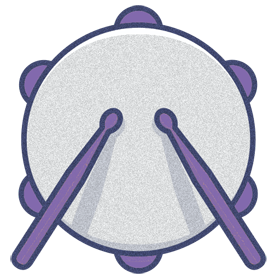# IQ Test Labs

Practice questions > Mathematics• We all require some numerical skills in our lives, whether it is to calculate our weekly shopping bill or to budget our income.
• Mathematical intelligence represents your ability to reason with numbers and to calculate basic arithmetic computations.
• Manipulating equations and understanding geometric shapes can be enhanced with improved mathematics skills.
• Mathematical intelligence is a strong indicator of general intelligence. Even simple everyday tasks require arithmetical operations even though numbers may not be involved.

#### Easy• Answered correctly by most people.
• Number sequences, probability, and arithmetic.

#### Medium• Answered correctly by more than half the people.
• Arithmetic, matrices and permutations.

#### Difficult• Answered correctly by less than half the people.
• Combinations, algebra, and number sequences.

### widgets Latest post

#### Get new questions to hone your IQ!### whatshotIn the news

#### Keeping the beat### pie_chartPolls

#### Quotations